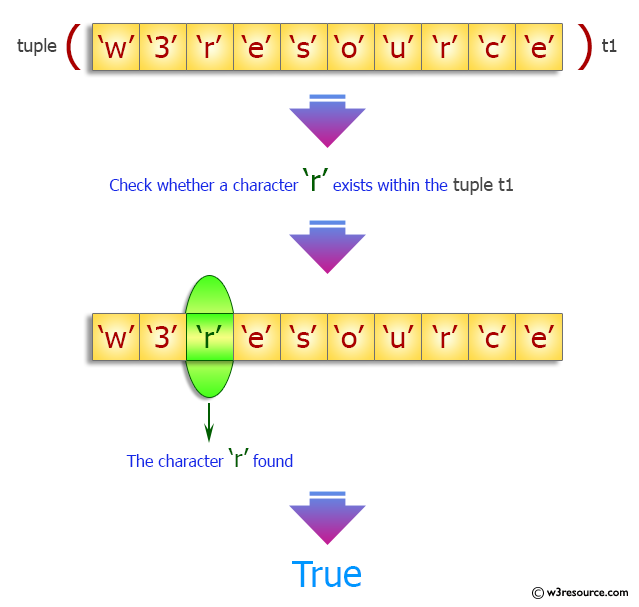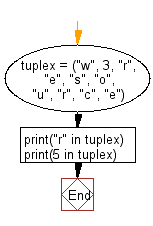﻿ Python: Check whether an element exists within a tuple - w3resource# Python Exercise: Check whether an element exists within a tuple

## Python tuple: Exercise-10 with Solution

Write a Python program to check whether an element exists within a tuple.

Sample Solution:-

Python Code:

``````tuplex = ("w", 3, "r", "e", "s", "o", "u", "r", "c", "e")
print("r" in tuplex)
print(5 in tuplex)
```
```

Sample Output:

```True
False
```

Pictorial Presentation:Flowchart:## Visualize Python code execution:

The following tool visualize what the computer is doing step-by-step as it executes the said program:

Python Code Editor:

Have another way to solve this solution? Contribute your code (and comments) through Disqus.

What is the difficulty level of this exercise?

Test your Programming skills with w3resource's quiz.

﻿

## Python: Tips of the Day

Decapitalizes the first letter of a string:

Example:

```def tips_decapitalize(s, upper_rest=False):
return s[:1].lower() + (s[1:].upper() if upper_rest else s[1:])
print(tips_decapitalize('PythonTips'))
print(tips_decapitalize('PythonTips', True))
```

Output:

```pythonTips
pYTHONTIPS
```